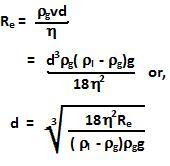Saturday, September 12, 2009

Irodov Problem 1.338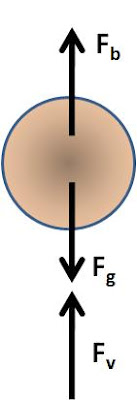Let the density of the fluid beand the density of the sphere be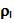and let the viscosity of the fluid be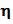. Let the terminal velocity of the sphere in the fluid be v.

As the sphere falls through the fluid there are three forces acting on it, i) the force of gravity, ii) the buoyant force due to the fluidand iii) the viscous force due to fluid on the sphere is given by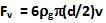. Since the body is moving at terminal velocity and there is no acceleration, all these forces must cancel out. Hence, we have,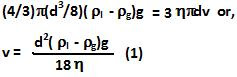The Reynold's number for a sphere moving in fluid is given by,